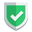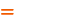我们相信：世界是美好的，你是我也是。平行空间的世界里面，不同版本的生活也在继续...

## 准备数据

``````let express = require("express")
let app = express();
let server = app.listen(3222, function () {
console.log(`访问地址为 http://localhost:\${port}`);
});``````

## 方案一，直接配置静态目录

``app.use(express.static('wwwroot')); // index.html 放在这里了``

## 方案二，配置路由处理页面请求

### 处理GET请求

``````let path = require("path");
let root = path.join(process.cwd(), "wwwroot");
app.get("/index.html", function (req, res) {
res.sendFile(path.join(root, "form.html"));
});``````

### 通配符匹配多页面

``````let path = require("path");
let root = path.join(process.cwd(), "wwwroot");
app.get("/:name.html", function (req, res) {
let file = path.join(root, "folder", req.params.name + ".html");
console.log(file, req.params.name);
res.sendFile(file);
});``````

### 模版处理

``````let path = require("path");
let fs = require('fs');
let root = path.join(process.cwd(), "wwwroot");
app.get("/:name.html", function (req, res) {
let file = path.join(root, "folder", req.params.name + ".html");
if (err) {
console.log(err);
data = "";
}
else {
data = data.toString('utf-8'); //buffer->string
}
data = data.replace(/苏南/gm, "sunan");
res.send(data);
});
});``````

## 结束语如果本文对您有帮助，或者节约了您的时间，欢迎打赏瓶饮料，建立下友谊关系。本博客不欢迎：各种镜像采集行为。请尊重原创文章内容，转载请保留作者链接。【福利】 腾讯云最新爆款活动！1核2G云服务器首年50元！【源码】本文代码片段及相关软件，请点此获取更多信息【绝密】秘籍文章入口，仅传授于有缘之人node    express